# The Metabigyrate Rhombicosidodecahedron

The metabigyrate rhombicosidodecahedron is the 74th Johnson solid, or J74 for short. It has 62 faces (12 pentagons, 30 squares, 20 triangles), 120 edges, and 60 vertices, just like the rhombicosidodecahedron, except that two oblique pentagonal cupola (J5) segments have been gyrated so that the polyhedron is no longer vertex-transitive.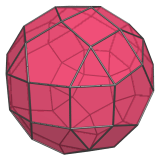J74 closely resembles the uniform rhombicosidodecahedron, but, just like J72, it can be recognized by noting the tell-tale pairs of adjacent squares and the triangles that share an edge with a pentagon: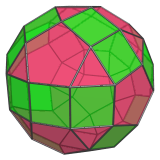Most notably, J74, like the other gyrates of the rhombicosidodecahedron involving non-opposite pentagonal cupola segments, have strips of 3 adjacent squares sharing edges. This feature is unique to these rhombicosidodecahedron modifications, namely, the metabigyrates and the trigyrate.

The uniform rhombicosidodecahedron does not have square faces that share edges with each other, nor does it have triangles that share edges with pentagons. These features arise from the gyrated configuration of the pentagonal cupola segments, highlighted below: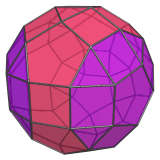## Projections

Here are some views of the metabigyrate rhombicosidodecahedron from various angles:

Projection Description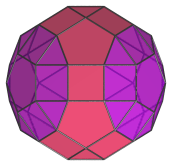Top view.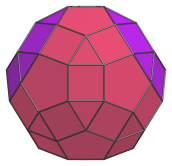Front view, parallel to pair of opposite triangles.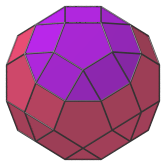Side view.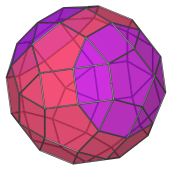Oblique view.

## Coordinates

The Cartesian coordinates of the metabigyrate rhombicosidodecahedron with edge length 2 are:

• (0, ±(φ+2), ±φ2)
• (±(φ+2), ±φ2, 0)
• (±φ2, 0, −(φ+2))
• (±1, ±1, ±φ3)
• (±1, ±φ3, ±1)
• (±φ3, ±1, −1)
• (±φ, ±φ2, ±2φ)
• (±φ2, ±2φ, ±φ)
• (±2φ, ±φ, −φ2)
• (±(8φ+1)/5, ±1, (6φ+7)/5)
• (±(8φ+6)/5, ±φ, (7+φ)/5)
• (±(13φ+1)/5, 0, (φ+2)/5)

where φ=(1+√5)/2 is the Golden Ratio.

Last updated 05 Apr 2019.